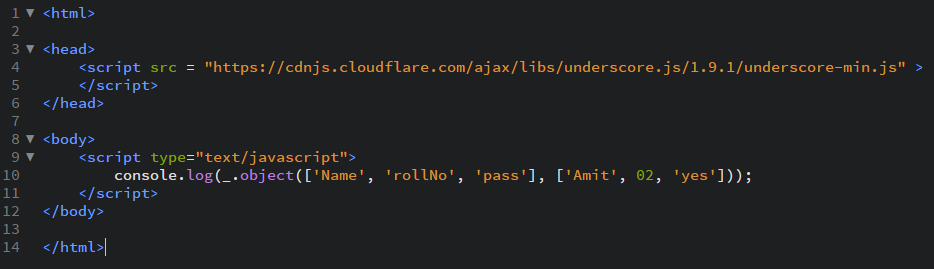GFG App
Open AppBrowser
Continue

# Underscore.js _.object() Function

_.object() function:

• It converts the array elements into objects.
• We can either pass all keys and then all the values or we can pass the key along with their value in pairs.
• It is used if we have more than one array but we want to make/ form a relation between these arrays.
• Also, size of the arrays is not necessary to be known before hand.

Syntax:

`_.object(list, [values])`

Parameters:
It takes two arguments:

• The list
• The values

Return value:
It returns an array having list of pairs from the keys and values passed.

Examples:

1. Passing 2 lists to _.object() function:
The ._object() function takes the element from the list one by one and makes it as an object with the value given in the other list. It takes the first elements of both the arrays and make them a single object by taking first array’s elements as the key and the other array’s as value. It will work only on first 2 arrays passed even if we pass a third array.

 `` `<``html``> ` `  `  `<``head``> ` `    ``<``script` `src` `=  ` `"https://cdnjs.cloudflare.com/ajax/libs/underscore.js/1.9.1/underscore-min.js"` `> ` `    `` ` ` ` `  `  `<``body``> ` `    ``<``script` `type``=``"text/javascript"``> ` `        ``console.log(_.object(['Akash', 'Amit', 'Aviral'], [01, 02, 03])); ` `    `` ` ` ` `  `  ` `

Output: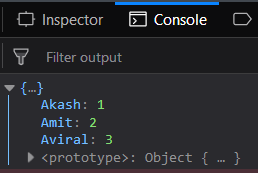2. Passing key along with it’s value in a single list to _.object() function:
The ._object() function takes the element from the list one by one and makes it as an object with the value given along with it in the same list. It takes all the elements in the first array and then form it a single object. It treats first array passed as a single object. We can pass n number of lists in this function.

 `` `<``html``> ` `  `  `<``head``> ` `    ``<``script` `src` `=  ` `"https://cdnjs.cloudflare.com/ajax/libs/underscore.js/1.9.1/underscore-min.js"` `> ` `    `` ` ` ` `  `  `<``body``> ` `    ``<``script` `type``=``"text/javascript"``> ` `        ``console.log(_.object([['Akash', 'Amit'], [01, 02], ['pass', 'pass']])); ` `    `` ` ` ` `  `  ` `

Output: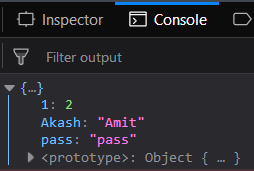3. Using _.object() function in real life
In this we use the second type of syntax mentioned above. If we want to obtain a database of a student named Amit (here) so we can just pass the parameters like his name, roll number and his result along with his details like his roll number is 2 and he is passed to the next class.

 `` `<``html``> ` `  `  `<``head``> ` `    ``<``script` `src` `=  ` `"https://cdnjs.cloudflare.com/ajax/libs/underscore.js/1.9.1/underscore-min.js"` `> ` `    `` ` ` ` `  `  `<``body``> ` `    ``<``script` `type``=``"text/javascript"``> ` `        ``console.log(_.object([['Name', 'Amit'], ['rollNo', 02], ['pass', 'yes']])); ` `    `` ` ` ` `  `  ` `

Output: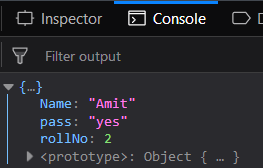4. Another way to obtain the same result as in example third using _.object() function:
In this also the result obtains will be same but the method of passing the key and value is different like here we pass all the keys in one array. Then, in the other array we pass all the values. Then the first element of the first array forms the first key and the first element of the second element of the second array as the value.

 `` `<``html``> ` `  `  `<``head``> ` `    ``<``script` `src` `=  ` `"https://cdnjs.cloudflare.com/ajax/libs/underscore.js/1.9.1/underscore-min.js"` `> ` `    `` ` ` ` `  `  `<``body``> ` `    ``<``script` `type``=``"text/javascript"``> ` `        ``console.log(_.object(['Name', 'rollNo', 'pass'], ['Amit', 02, 'yes'])); ` `    `` ` ` ` `  `  ` `

Output: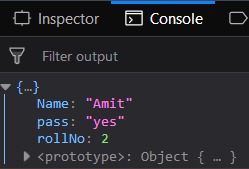NOTE:
These commands will not work in Google console or in firefox as for these additional files need to be added which they didn’t have added.
 `` `<``script` `type``=``"text/javascript"`  `src` `=``"https://cdnjs.cloudflare.com/ajax/libs/underscore.js/1.9.1/underscore-min.js"``> ` ` `